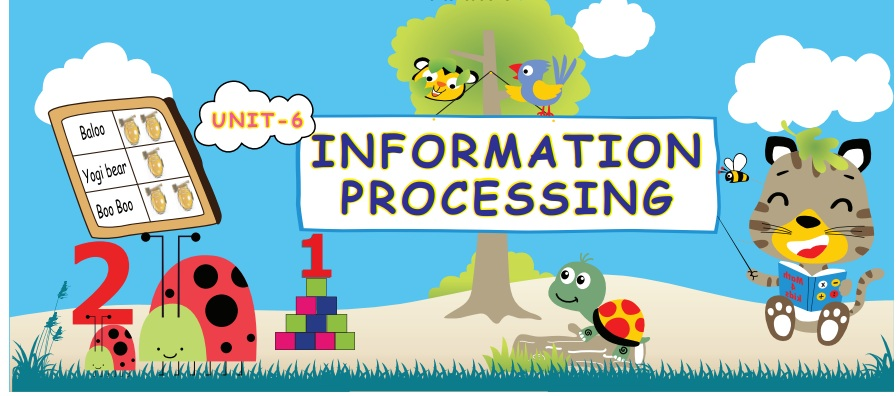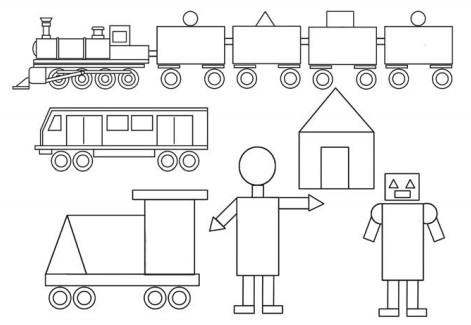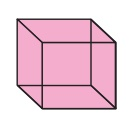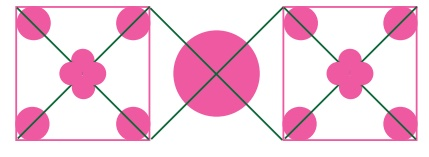Home | | Maths 5th Std | Modelling

# Modelling

A way of introducing the mathematical concept. Modelling is an activity like drawings as sketches, Physical models, computer programmes or mathematical formulas. Let us see the following models using the different shapes such as circles, rectangles and triangles.

UNIT−6

INFORMATION PROCESSINGModelling

A way of introducing the mathematical concept.

Modelling is an activity like drawings as sketches, Physical models, computer programmes or mathematical formulas.

Let us see the following models using the different shapes such as circles, rectangles and triangles.

Geometrical shaped objects.Let us see artistic chains with different coloured beads.Try this

How many squares are there?ActivityActivity

The following shapes are drawn instead of numbers 0, 1, 2, 3, 4, 5. Shall we find the number of each shapes.Let us know

In our street,

There are two times Cats of Dogs

D = Dog C − Cat

Activity

Complete the followingNumber of circles - 17

Number of Triangles - 18

Number of Squares - 2

Try this

How many rectangles are there in this diagram?There are 24 rectangles in this diagram.

Rectangles form are,

ACGE, EGLJ, JLOM, CDPO, ACLJ, EGOM, ACOM, ADPM, ABNM, BCPN, BDPN, ABFE, EFKJ, EF1H, JKNM, BCGF, FGLK, EFNM, KLON, BCLK, FGON, ABIH, HIKJ, HINM

Example

Birthday triangle Cake

The given figure is a triangle shaped birthday cake, this was bought by the parents to celebrate the birthday of a three year child. There are six flowers in the cake, you have to divide this cake into three equal parts so as to have two flowers in each part. There should not be more than three cuts.Tags : Information Processing | Term 2 Chapter 6 | 5th Maths , 5th Maths : Term 2 Unit 6 : Information Processing
Study Material, Lecturing Notes, Assignment, Reference, Wiki description explanation, brief detail
5th Maths : Term 2 Unit 6 : Information Processing : Modelling | Information Processing | Term 2 Chapter 6 | 5th Maths